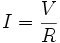## 2007/06/14

### Ohm's Law Basic

Ohm's law states that, in an electrical circuit, the current passing through a conductor from one terminal point on the conductor to another terminal point on the conductor is directly proportional to the potential difference(i.e. voltage drop or voltage) across the two terminal points and inversely proportional to the resistance of the conductor between the two terminal points. In mathematical terms, this is written as:where I is the current, V is the potential difference, and R is a constant called the resistance. The potential difference is also known as the voltage drop , and is sometimes denoted by E or U instead of V. 
The SI unit of current is the ampere
; that of potential difference is the volt ; and that of resistance is the ohm, equal to one volt per ampereA voltage source, V, drives an electric current, I , through resistor, R, the three quantities obeying Ohm's law: V = IR.

Sample RAE question:-Picture 1

i- Follow the picture 1. Given I = 1 amp and V = 10 volt. Calculate the resistor ?

V = IR or V = I x R

so calculate the resistor using this formula

R = V / I
R = 10 volt / 1 amp
R = 10 ohm.

ii- If the resistor R = 20 ohm. Calculate the voltage ?. Given I = 4 amp.